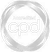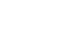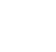# Portfolio Volatility### Abdulla Javeri

In this video, Abdulla outlines the importance of monitoring portfolio volatility risk and the way it is calculated for a two asset portfolio, and a three stock portfolio.

Financial MathsIn this video, Abdulla outlines the importance of monitoring portfolio volatility risk and the way it is calculated for a two asset portfolio, and a three stock portfolio.

Speak to an expert

Speak to an expert today to access this and all of the content on our platform.### Portfolio Volatility

5 mins 39 secs

Overview

Volatilities and correlations are not static, they can change, sometimes very suddenly, therefore the risk in a portfolio can change over time, or sometimes very suddenly. It’s a case of constantly monitoring the risks, even perhaps trying to anticipate those changes in advance and making appropriate adjustments.

Key learning objectives:

• Learn to calculate the volatility of a two asset portfolio

• Outline the formula used for a three stock portfolio

• Outline the uses of portfolio volatility in risk management

## Speak to an expert

Speak to an expert today to access this and all of the content on our platform.

Summary

#### What is volatility?

Volatility is usually described as one standard deviation of annual percentage price movements and is a measure of risk, the higher the volatility, the higher the risk.

#### Why is there a trade-off between risk and return?

• Holding high risk assets provides the potential for greater returns, but we also have to accept a risk of potentially large losses.
• If we were risk averse, we would invest in assets that have low risk, in other words, those less volatile. However, in this case, we’re unlikely to make large returns.

#### How can we calculate the volatility of a two asset portfolio?This formula involves three key components:

1. The volatility of each individual asset
2. The weights of the assets as a proportion of the portfolio value
3. The correlations between the assets

#### Using the information provided in the table below, what are the relevant volatilities?

 Stock Holding Price Value Weight Volatility Correlation A 1000 100 100,000 50% 35% 1.00 B 1000 100 100,000 50% 35%
• Portfolio Value = 200,000
• Average Volatility = 35%
• Weighted Volatility = 35%
• Portfolio Volatility = 35%

#### What would happen if the two stocks had a perfectly negative correlation (-1)?

If one goes up, the other comes down by exactly the same amount. Using our existing numbers, the value of the portfolio would remain unchanged, as a price of one would be offset by the change of the other. In other words, the portfolio volatility would be zero - 0%.

#### What could we do If we wanted to reduce the volatility of a portfolio?

1. Introduce a less volatile stock, change the volatility for stock a to say, 20%
2. Increase the weight of stock A
3. If we could find uncorrelated, or negatively correlated stocks, we could reduce it further

#### What is the effect on the portfolio volatility if the volatility for stock A was reduced to 20%?

 Stock Holding Price Value Weight Volatility Correlation A 1000 100 100,000 50% 20% 1.00 B 1000 100 100,000 50% 35%
• Portfolio Value = 200,000
• Average Volatility = 27.50%
• Weighted Volatility = 27.50%
• Portfolio Volatility = 27.50%

#### What is the effect on the relevant volatilities if the weight of stock A was increased to 1500?

 Stock Holding Price Value Weight Volatility Correlation A 1500 100 150,000 60% 20% 1.00 B 1000 100 100,000 40% 35%
• Portfolio Value = 250,000
• Average Volatility = 27.50%
• Weighted Volatility = 26.00%
• Portfolio Volatility = 26.00%

#### What is the effect on the relevant volatilities if the correlation was 0?

 Stock Holding Price Value Weight Volatility Correlation A 1500 100 150,000 75% 20% 0 B 500 100 50,000 25% 35%
• Portfolio Value = 200,000
• Average Volatility = 27.50%
• Weighted Volatility = 23.75%
• Portfolio Volatility = 17.37%

#### What is the effect on the relevant volatilities if the correlation was -0.7?

 Stock Holding Price Value Weight Volatility Correlation A 1500 100 150,000 75% 20% -0.7 B 500 100 50,000 25% 35%
• Portfolio Value = 200,000
• Average Volatility = 27.50%
• Weighted Volatility = 23.75%
• Portfolio Volatility = 10.85%

#### What is the formula used for a three stock portfolio?#### What are the uses of portfolio volatility in risk management?

• The amount of risk associated with asset holdings can be calculated as a whole
• It can reduce the amount of capital that needs to be held against potential losses in the value of those assets.

## Speak to an expert

Speak to an expert today to access this and all of the content on our platform.### Abdulla Javeri

Abdulla’s career in the financial markets started in 1990 when he entered the trading floor of the London International Financial Futures Exchange, LIFFE, and qualified as a pit trader in equity and equity index options. In 1996, Abdulla became a trainer for regulatory qualifications and then for non-exam courses, primarily covering all major financial products.

There are no available videos from "Abdulla Javeri"

### Accredited by